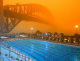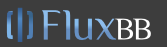# Math Is Fun Forum

Discussion about math, puzzles, games and fun.   Useful symbols: ÷ × ½ √ ∞ ≠ ≤ ≥ ≈ ⇒ ± ∈ Δ θ ∴ ∑ ∫  π  -¹ ² ³ °

You are not logged in.

## #1 2023-09-27 02:12:13

sologuitar
Member
Registered: 2022-09-19
Posts: 467

### More Factor Completely

Factor completely.

5 + 16x - 16x^2

Let me see.

5(-16) = -80

What two numbers when multiplied yield -80 but when added produce 16?

20(-4) = -80

20 + (-4) = 16

Yes, 20 and -4 will do.

Put it all together to force factor by grouping.

5 + 20x - 4x - 16x^2

Factor by grouping.

5 + 20x = 5(1 + 4x)...Group A

-4x - 16x^2 = -4x(1 + 4x)...Group B

Put both groups together.

5(1 + 4x) - 4x(1 + 4x)

My answer: (5 - 4x)(1 + 4x)

Book's answer: -(4x - 5)(4x + 1)

What did I do wrong?

Offline

## #2 2023-09-27 02:40:07

Jai GaneshRegistered: 2005-06-28
Posts: 44,425

### Re: More Factor Completely

The book answer is -(4x - 5)(4x + 1) which is the same as (5 - 4x)(4x + 1).

It appears to me that if one wants to make progress in mathematics, one should study the masters and not the pupils. - Niels Henrik Abel.

Nothing is better than reading and gaining more and more knowledge - Stephen William Hawking.

Offline

## #3 2023-09-27 02:54:21

sologuitar
Member
Registered: 2022-09-19
Posts: 467

### Re: More Factor Completely

Ok. Thanks. Now, I am having a hard uploading images to the site.

Here is a question about Even, Odd ,Neither.

The image is found here:

https://imgur.com/a/hQDSFH4

Offline

## #4 2023-09-27 04:22:30

BobRegistered: 2010-06-20
Posts: 9,863

### Re: More Factor Completely

A function is said to be even if it is symmetrical in the y axis.  Here are some examples:

y = x^2

y = x^4

y = x^6 + x^4 - x^2

From these examples you can probably see why these are called even functions.

To test algebraically replace every x in the function with -x.  If you get the same function back, then it's even.

example:

y = x^2 Replace x with -x  .... ynew = (-x)^2 = x^2 = y. So y is even.

In a similar way odd functions have rotational symmetry around the origin order 2. ie. If you rotate the graph 180 around (0,0) and the graph looks the same then it is a odd function.

example:

y = x^3

But most likely, a function is neither The second example looks like y = e^x and this is neither odd nor even.

If you track left on the graph, as x tends to minus infinity y tends to zero. There are no negative y values. As you track right, as x tends to + infinity y tends to + infinity.  So the range is (0, + infinity). The round brackets indicate that the endpoints are never achieved.

Bob

Children are not defined by school ...........The Fonz
You cannot teach a man anything;  you can only help him find it within himself..........Galileo Galilei
Sometimes I deliberately make mistakes, just to test you!  …………….BobOffline

## #5 2023-09-27 06:46:29

sologuitar
Member
Registered: 2022-09-19
Posts: 467

### Re: More Factor Completely

Bob wrote:

A function is said to be even if it is symmetrical in the y axis.  Here are some examples:

y = x^2

y = x^4

y = x^6 + x^4 - x^2

From these examples you can probably see why these are called even functions.

To test algebraically replace every x in the function with -x.  If you get the same function back, then it's even.

example:

y = x^2 Replace x with -x  .... ynew = (-x)^2 = x^2 = y. So y is even.

In a similar way odd functions have rotational symmetry around the origin order 2. ie. If you rotate the graph 180 around (0,0) and the graph looks the same then it is a odd function.

example:

y = x^3

But most likely, a function is neither The second example looks like y = e^x and this is neither odd nor even.

If you track left on the graph, as x tends to minus infinity y tends to zero. There are no negative y values. As you track right, as x tends to + infinity y tends to + infinity.  So the range is (0, + infinity). The round brackets indicate that the endpoints are never achieved.

Bob

Did you see the photo? How did you see the photo? I cannot figure out how to upload photos. As long as you can see my URL photo, we are in business. I like your explanation about even, odd and neither functions. Thank you.

Offline

## #6 2023-09-27 06:51:31

amnkb
Member
Registered: 2023-09-19
Posts: 175

### Re: More Factor Completely

harpazo1965 wrote:

Ok. Thanks. Now, I am having a hard uploading images to the site.

Here is a question about Even, Odd ,Neither.

The image is found here:putting in img tags doesnt work

a. i think the 1st fcn is y = 1/x (imnshp, when they don't give you fcn its kind of unfair to tell you to find stuff out about it)

"even" means it matches on both sides of the y axis

"odd" means it matches on both sides of diagonal y = x

this fcn is odd because you can fold graph on diagonal and teh 2 halfs will match

domain is x not equal to 0 (im guessing from the graph; it looks like there is a vertical asymptiote at x=0)

range is y not equal to 0 (im guessing from the graphl it looks like there is a horizontal asymptote at y=0)

b. it looks like maybe an exponential, like y=e^x ?

if you fold the graph on the y axis the halfs wont match so its not even

if you fold on the diagonal the halfs wont match so its not odd

domain looks like all x

i dont know how your supposed to know the range from the picture; the graph could cross the axis off to the left
but if you guess that the graph close to the center tells you everything you need to know  then it looks like graph is always above x axis

Offline

## #7 2023-09-27 07:05:48

sologuitar
Member
Registered: 2022-09-19
Posts: 467

### Re: More Factor Completely

amnkb wrote:
harpazo1965 wrote:

Ok. Thanks. Now, I am having a hard uploading images to the site.

Here is a question about Even, Odd ,Neither.

The image is found here:

https://imgur.com/a/hQDSFH4

putting in img tags doesnt work

a. i think the 1st fcn is y = 1/x (imnshp, when they don't give you fcn its kind of unfair to tell you to find stuff out about it)

"even" means it matches on both sides of the y axis

"odd" means it matches on both sides of diagonal y = x

this fcn is odd because you can fold graph on diagonal and teh 2 halfs will match

domain is x not equal to 0 (im guessing from the graph; it looks like there is a vertical asymptiote at x=0)

range is y not equal to 0 (im guessing from the graphl it looks like there is a horizontal asymptote at y=0)

b. it looks like maybe an exponential, like y=e^x ?

if you fold the graph on the y axis the halfs wont match so its not even

if you fold on the diagonal the halfs wont match so its not odd

domain looks like all x

i dont know how your supposed to know the range from the picture; the graph could cross the axis off to the left
but if you guess that the graph close to the center tells you everything you need to know  then it looks like graph is always above x axis

Bob saw my photo. This is what matters.

Offline

## #8 2023-09-27 15:23:14

phrontister
Real MemberFrom: The Land of Tomorrow
Registered: 2009-07-12
Posts: 4,793

### Re: More Factor Completely

Hi amnkb,

amnkb wrote:

putting in img tags doesnt work

For an image to display on this forum, the link must be to the actual image file: ie, it has to contain an 'image extension' at the end of the filename (such as '.jpg' or '.png')...as per the BBCode example below.

The OP's link is an Imgur webpage url, and hence no image will display here.

The webpage link from Post #3 (https://imgur.com/a/hQDSFH4) gives me a '404' error. Maybe the link worked for Bob initially, but if it did, I wonder if it still does. I only tried it today...

The following instruction and example are from the BBCode instruction page that you can access by clicking on the 'BBCode' button under the Message box into which you type a post (or by clicking here: BBCode), and then scrolling to the bottom of the 'Links and Images' box:

``````If you want to display an image you can use the img tag. The text appearing after the "=" sign in the opening tag is used for the alt attribute and should be included whenever possible.
[img=FluxBB bbcode test]http://www.mathisfunforum.com/img/test.png[/img]``````

That code producesLast edited by phrontister (2023-09-27 18:58:26)

"The good news about computers is that they do what you tell them to do. The bad news is that they do what you tell them to do." - Ted Nelson

Offline

## #9 2023-09-27 20:23:02

BobRegistered: 2010-06-20
Posts: 9,863

### Re: More Factor Completely

amnkb wrote:

"odd" means it matches on both sides of diagonal y = x

**

That's not the definition I have always used.

https://www.mathsisfun.com/algebra/func … -even.html

The url in post 3 worked when I made my post but not now.  Maybe the poster removed it from imgur ???

There were two graphs. The first was y = x^2 or something similar. The second was, I think, y = e^x or some thing similar. There were no scales so the exact function could not be determined but enough to show the symmetry or not.

Bob

ps ** If a graph of a function is reflected in the line y=x this is equivalent to interchanging y and x and the resulting graph shows the inverse function.

eg.  Compare y = 2x + 3 and y = (x-3)/2

Children are not defined by school ...........The Fonz
You cannot teach a man anything;  you can only help him find it within himself..........Galileo Galilei
Sometimes I deliberately make mistakes, just to test you!  …………….BobOffline

## #10 2023-09-28 03:54:53

sologuitar
Member
Registered: 2022-09-19
Posts: 467

### Re: More Factor Completely

Bob wrote:
amnkb wrote:

"odd" means it matches on both sides of diagonal y = x

**

That's not the definition I have always used.

https://www.mathsisfun.com/algebra/func … -even.html

The url in post 3 worked when I made my post but not now.  Maybe the poster removed it from imgur ???

There were two graphs. The first was y = x^2 or something similar. The second was, I think, y = e^x or some thing similar. There were no scales so the exact function could not be determined but enough to show the symmetry or not.

Bob

ps ** If a graph of a function is reflected in the line y=x this is equivalent to interchanging y and x and the resulting graph shows the inverse function.

eg.  Compare y = 2x + 3 and y = (x-3)/2

Hi Bob.

Yes, I removed the photo from imgur. I will post several questions later showing my work or at least effort. I need all the practice I can get with imgur.
I am not savvy in terms of computers, laptops, tablets, and cell phones. I just know internet basics. Again, I deleted all imgur uploads yesterday. I will keep my uploaded stuff there moving forward.

Offline